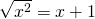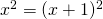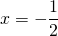# Solution assignment 10 Root equations

### Assignment 10

Solve:### Solution

We can first try to write the conditions which must be met for which this equation is defined. We can also begin solving the equation and check afterwards whether solutions satisfy the equation. We now choose the second approach.
We square both sides of the equation:After some calculations we get the solution:which satisfies the equation.